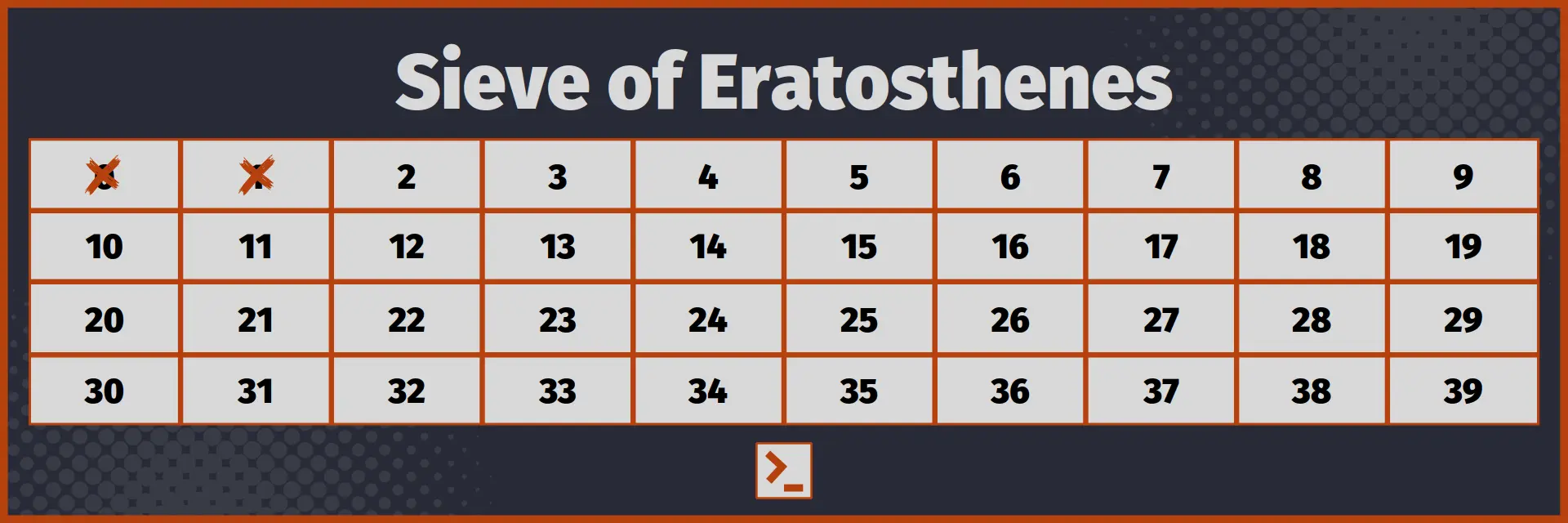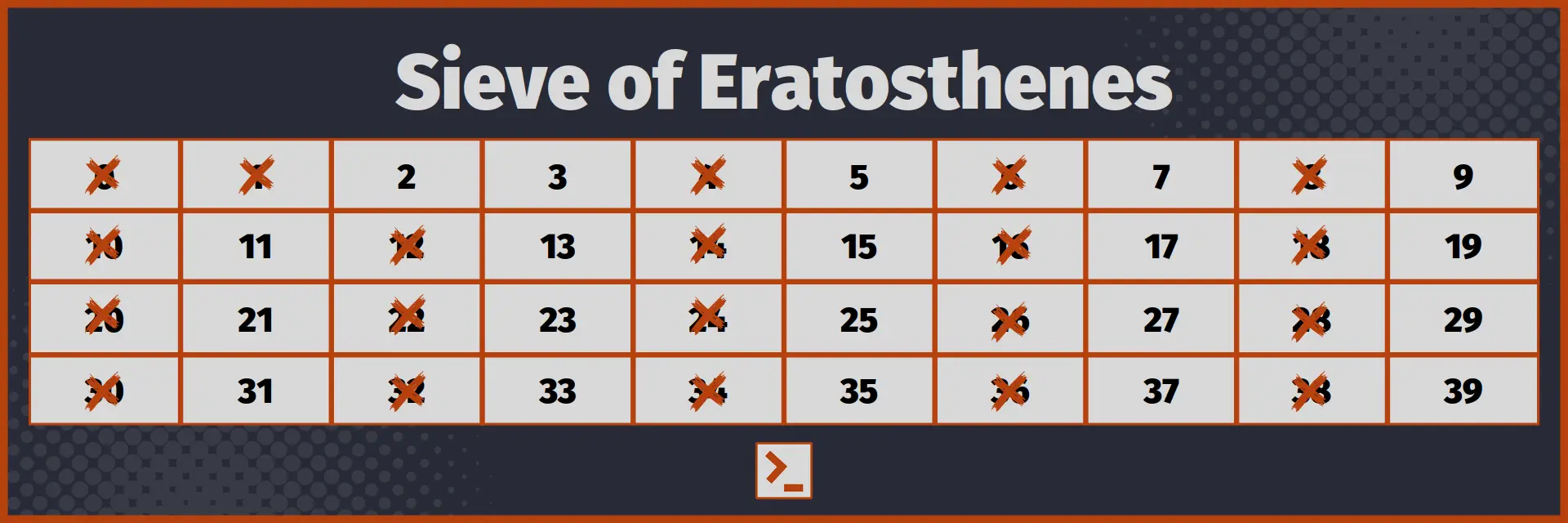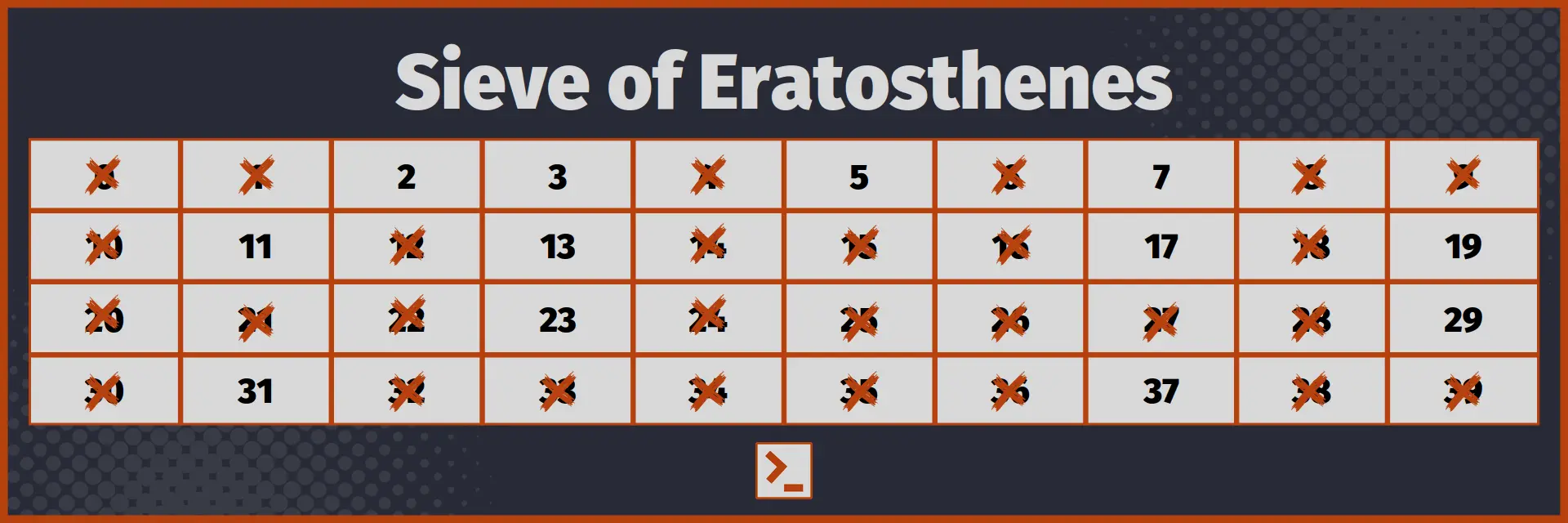# Sieve of Eratosthenes

and program to find all the prime numbers upto a given number in Rust Language.

## Introduction

Sieve of Eratosthenes is one of the most efficient algorithm for finding all the prime numbers upto a given number.

It can print all the prime numbers upto a given number in nearly linear time complexity , O( N log(log( N ))) to be precise, and linear space complexity.

It also generates a complete list, which number upto N are primes and which are not. We can now check if a given number is prime or not in constant time complexity !

Sounds interesting? It is simple enough to understand also.

## Algorithm

In Sieve of Eratosthenes, we will be storing if a number is prime or not at the index. So, to check if 5 is prime, we just have to check for value at array, if it is true or false.

1. Initially, take a boolean array / vector of size n+1 and set initially every element to true. Now, set 0 and 1 to false ( Obviously number 0 and 1 are not prime numbers. )Initial Array

1. Now, traverse from 2 to n, and if the number is marked as true, mark all its multiple as false, except the number itself. Because if obviously, a number is multiple of a number greater than or equal to 2, it is not prime. Also, if a number is marked true, it must be prime because it was not marked as False by any smaller number. Therefore, it is not a multiple of any smaller number, hence it is prime number.After marking false all the multiples of 2

1. If any number, say x, is marked as false, there is no need to traverse through its multiples, because the multiples of x will be already be marked as false by the least prime number that divides x.

2. Repeat step 2 and 3 for all numbers upto n

3. After traversing all the numbers, we are left with a boolean array / vector in which the i index is set true, if number is prime, and false otherwise.Final Array

I hope you understood the Algorithm and liked it. Now, let us implement it.

## Program without optimization

Below is the basic implementation of Sieve of Eratosthenes.

```fn sieve(n: usize) -> Vec<bool>{
// Initialize Sieve Array with all elements initially set to True    let mut sieve_array = vec![true; n+1];
// Set arr and arr to false, because 0 and 1 are not prime    sieve_array =  false;    sieve_array = false;
// Traverse from 2 to n    // If a number is prime, mark all its multiples except number itself as false    for i in 2..n+1 {        if sieve_array[i] {
// Mark all the multiples except number itself as false            for j in (2*i..n+1).step_by(i) {                sieve_array[j] = false;            }        }    }
return sieve_array;}```

Program With driver code

```use std::io::stdin;
fn take_int() -> usize {    let mut input = String::new();    stdin().read_line(&mut input).unwrap();    return input.trim().parse().unwrap();}

fn sieve(n: usize) -> Vec<bool>{
// Initialize Sieve Array with all elements initially set to True    let mut sieve_array = vec![true; n+1];
// Set arr and arr to false, because 0 and 1 are not prime    sieve_array =  false;    sieve_array = false;
// Traverse from 2 to n    // If a number is prime, mark all its multiples except number itself as false    for i in 2..n+1 {        if sieve_array[i] {
// Mark all the multiples except number itself as false            for j in (2*i..n+1).step_by(i) {                sieve_array[j] = false;            }        }    }
return sieve_array;}
// Driver Codepub fn main() {    // Take input and print all the numbers upto number    // And tell if they are prime    let number = take_int();
// Generate a sieve array for the given number    let sieve_array = sieve(number);
// Output if the number is prime or not    // We can now tell whether a number less than equal to N    // is Prime or not in O( 1 ) or constant time complexity    for i in 2..number+1 {        if sieve_array[i] {            println!("Yes, {}  is a prime number", i);        }        else {            println!("No, {} is not a prime number", i);        }    }}```

Input

6

Output

Yes, 2 is a prime number
Yes, 3 is a prime number
No, 4 is not a prime number
Yes, 5 is a prime number
No, 6 is not a prime number

Time Complexity : O( n log(log(n)) )
Space Complexity : O( n )

## Optimized Sieve

1. If we observe carefully, we can see that if a number is marked as prime, its smallest multiple that is not already marked as prime is its square.
For example : for number 5,
5×1 = 5
5×2 = 10
5×3 = 15
5×4 = 20
5×5 = 25
We can see that all the numbers upto 25 will already be marked as non-prime by numbers smaller than 5, that is 2, 3, 4 and so on. So, we do not have to traverse them again.

2. We have to check numbers upto square root of N only, because if a number is not divisible by any number upto square root of N, it will not be divisible by any larger number.
We used the same logic to optimize code to Check whether a number is Prime or not

3. We have to check only odd numbers, because all even numbers except 2 are composite numbers. Similarly, we have to check only odd multiples of numbers ( except 2 ), because even multiples are already marked false by 2.

These optimizations can make Sieve method faster by more than 50% in general, though it do not affect the time complexity of the code.

Implementation of Sieve of Eratosthenes after these optimizations looks like

```// Optimized Sieve of Eratosthenesfn sieve(n: usize) -> Vec<bool>{
// Initialize Sieve Array with all elements initially set to True    let mut sieve_array = vec![true; n+1];
// Set arr and arr to false, because 0 and 1 are not prime    sieve_array =  false;    sieve_array = false;
// Mark all even numbers as false, except 2    for i in (4..n+1).step_by(2) {        sieve_array[i] = false;    }
// Traverse from 3 to square root of n    // If a number is prime, mark all its multiples except number itself as false    // Optimization : Check numbers only upto square root of n    let mut i = 3;    while i*i<= n+1 {        if sieve_array[i] {
// Mark all the multiples except number itself as false            // Optimization : start from i*i, because smaller multiples are already marked            // Optimization : use 2*i as step, because  we need to check only odd multiples            for j in (i*i..n+1).step_by(2*i) {                sieve_array[j] = false;            }        }        // We do not have to check even numbers.        // So, we increment i by 2        i+=2;    }
return sieve_array;}```

Use the same driver code.

Input

6

Output

Yes, 2 is a prime number
Yes, 3 is a prime number
No, 4 is not a prime number
Yes, 5 is a prime number
No, 6 is not a prime number

Time Complexity : O( n log(log(n)) )
Space Complexity : O( n )

## Conclusion

Sieve of Eratosthenes is a very popular and useful algorithm, used for finding all the prime numbers upto a given number. In this article, we understood the Sieve of Eratosthenes method and also wrote function to find all the primes numbers upto a given number in Rust.

Here is optimized function for easy access.

```fn sieve(n: usize) -> Vec<bool>{    let mut sieve_array = vec![true; n+1];    sieve_array =  false;    sieve_array = false;    for i in (4..n+1).step_by(2) { sieve_array[i] = false; }
let mut i = 3;    while i*i<= n+1 {        if sieve_array[i] {            for j in (i*i..n+1).step_by(2*i) { sieve_array[j] = false; }        }        i+=2;    }    return sieve_array;}```

Thank You